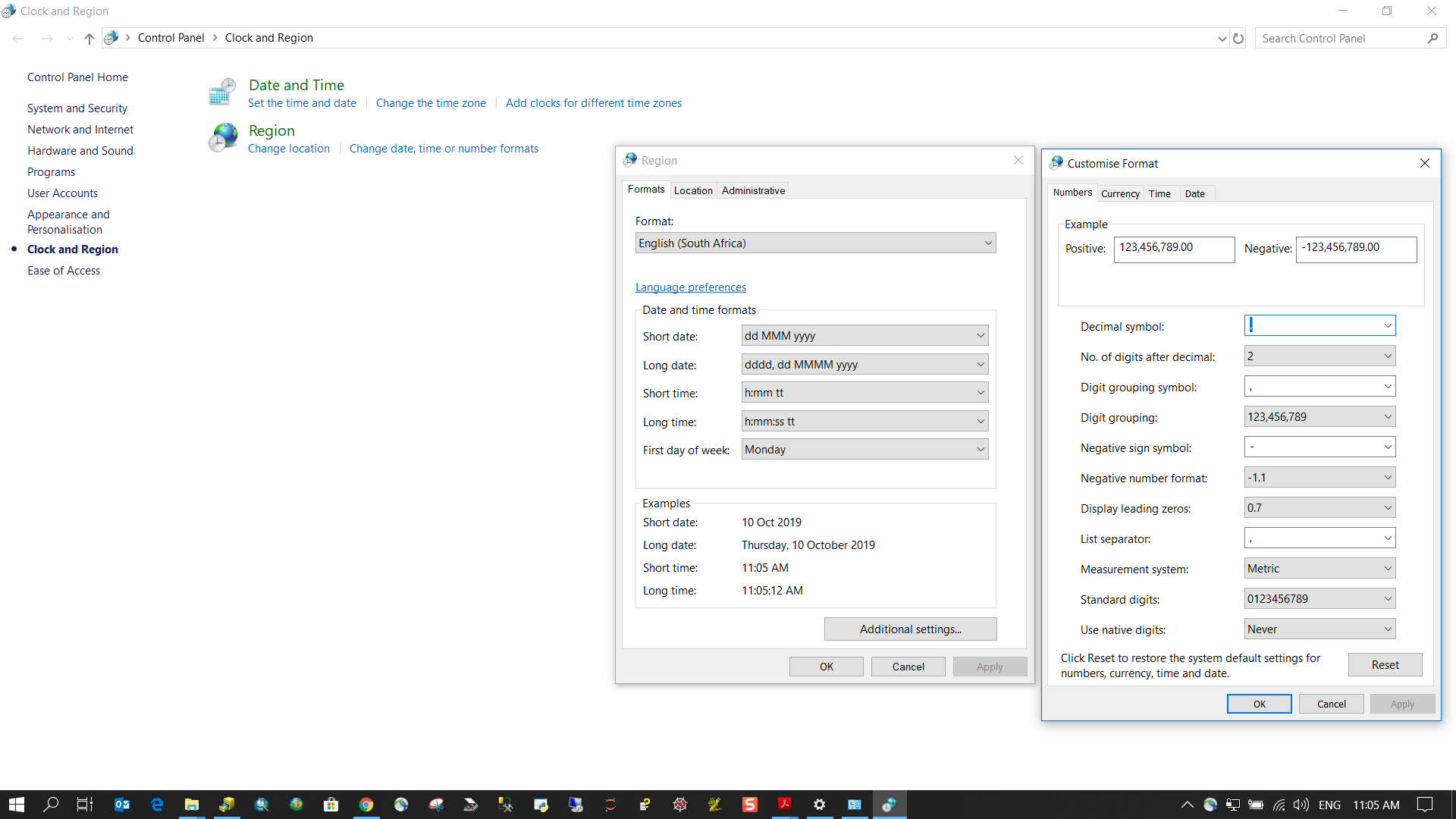# Python expression in Modelbuilder

215
5
Jump to solution
10-09-2019 12:34 AMNew Contributor

Hi,

I need to sum two fields which are in different layers...

Using Calculate field and running the expression sum(%Summe_Trassen_eigen%,%Summe_Trassen_angemietet%)

I get incorrect value.

sum(%Summe_Trassen_eigen%,%Summe_Trassen_angemietet%)

The example is: "sum([582,871017020542,2826,20859771853])

the result is: 891876795803 - whats wrong, it has to be 3409,0796...

can you advice whats wrong?

thanks

Tags (3)
1 Solution

Accepted SolutionsbyMVP Frequent Contributor

Changing the decimal symbol is buried quite deeply in the Time & Date setting of Windows.5 RepliesbyMVP Frequent Contributor

You appear to have your decimal delimiter (radix) set to a comma ",".

So:

582 +

871017020542 +

2826 +

20859771853

= 891876795803

So change it back to ".", a decimal point.New Contributor

Hi @Neil Ayres,

thanks for answer.

The problem is that I get this values from summstatistic (values are "double"). So it gives me automatic "comma" values. Is there a way how to change double to integer or how to change it to a decimal point?

"Summstatistic" > (the fields are double) > "Get field value" > (double, with comma) - *I do not need the values behind the commabyMVP Frequent Contributor

Changing the decimal symbol is buried quite deeply in the Time & Date setting of Windows.New Contributor

Great thanks a lot!byMVP Frequent Contributor

Sorry, those dialogs are under "Region"